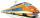# Triangle + motion problems - math problems

1. The swimmerThe swimmer swims at a constant speed of 0.85 m/s relative to water flow. The current speed in the river is 0.40 m/s, the river width is 90 m. a) What is the resulting speed of the swimmer with respect to the tree on the riverbank when the swimmer motioFrom the junction of two streets that are perpendicular to each other, two cyclists (each on another street) walked out. One ran 18 km/h and the second 24 km/h. How are they away from a) 6 minutes, b) 15 minutes?
3. Two aircraftTwo planes fly to the airport. At some point, the first airplane is away from the airport 98 km and the second 138 km. The first aircraft flies at an average speed of 420 km/h, the second average speed is 360 km/h, while the tracks of both planes are perp
4. TrainThe train is running at speeds of 96 km/h. From the beginning of braking to full stop train run for 3.3 minutes. If the train slows the braking equally, calculate the distance of the place from the station where you need to start to brake.
5. Rotation of the EarthCalculate the circumferential speed of the Earth's surface at a latitude of 61°​​. Consider a globe with a radius of 6378 km.

We apologize, but in this category are not a lot of examples.
Do you have an interesting mathematical word problem that you can't solve it? Enter it, and we can try to solve it.

We will send a solution to your e-mail address. Solved examples are also published here. Please enter the e-mail correctly and check whether you don't have a full mailbox.

Please do not submit problems from current active competitions such as Mathematical Olympiad, correspondence seminars etc...